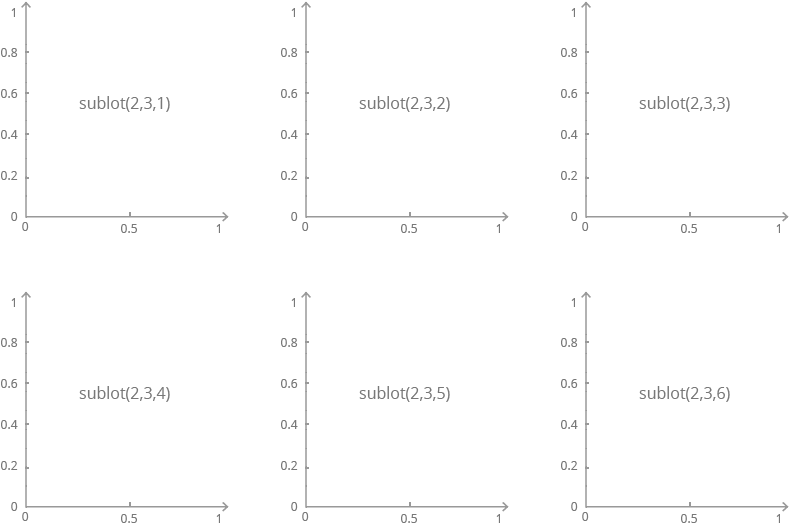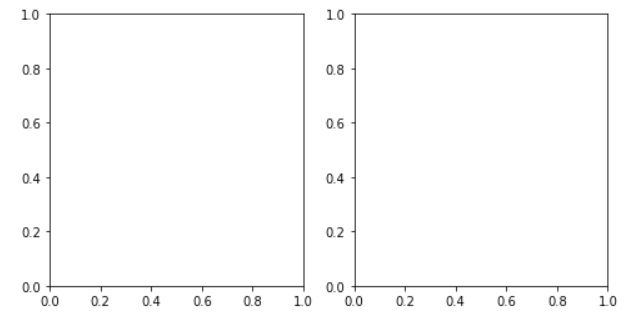Deals Of The Week - hours only!Up to 80% off on all courses and bundles.-Close
Introduction
Line plots
Multiple histograms
9. Creating multiple subplots
Other plot types
Summary

## Instruction

Great! First, we need to create a figure:

figure = plt.figure(figsize=(8, 4))

Previously, we simply created a subplot using plt.subplot(). This time, we'll use another method to add multiple subplots to the same figure:

subplot1 = figure.add_subplot(121)
subplot2 = figure.add_subplot(122)

You may be wondering about the mysterious numbers inside the parentheses – 121 and 122.

In general, add_suplot(xyn) (shorthand for add_suplot(x, y, n)) refers to a grid of subplots with x rows and y columns, and it creates a subplot at position n. Subplots are numbered from left to right in subsequent rows. For instance:Now, in our code:

• (121) is short for (1, 2, 1) and means: create a grid with 1 row and 2 columns, and place a subplot in the 1st column.
• (122) is short for (1, 2, 2) and means: create a grid with 1 row and 2 columns, and place a subplot in the 2nd column.

We will see the following result:## Exercise

Create a figure with dimensions 12x4, and add three subplots in a single row.

### Stuck? Here's a hint!

To add the first subplot, use:

subplot1 = figure.add_subplot(131)